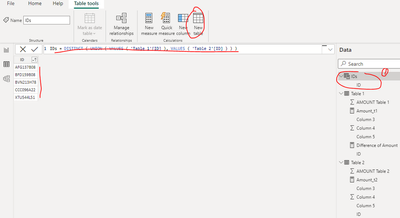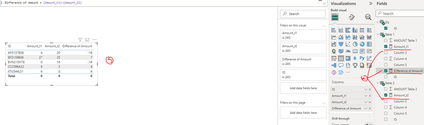cancel
Showing results for
Did you mean:Frequent Visitor

## Let measure show the correct total amount in a table

I have implemented the below based on a solution provided on this forum to show amounts from different tables as columns based on the union of IDs. However, the totals show zero for all columns based on the picture in step 3. How can I fix this?

1. Create a dimension table with IDs which is from both Table 1 and Table 2

`IDs = DISTINCT ( UNION ( VALUES ( 'Table 1'[ID] ), VALUES ( 'Table 2'[ID] ) ) )`2. Create the measures as below to get the sum of amount in Table 1 and Table 2, the difference of amount

```Amount_t1 =
VAR _selid =
SELECTEDVALUE ( 'IDs'[ID] )
RETURN
CALCULATE (
SUM ( 'Table 1'[AMOUNT Table 1] ),
FILTER ( 'Table 1', 'Table 1'[ID] = _selid )
) + 0```
```Amount_t2 =
VAR _selid =
SELECTEDVALUE ( 'IDs'[ID] )
RETURN
CALCULATE (
SUM ( 'Table 2'[AMOUNT Table 2] ),
FILTER ( 'Table 2', 'Table 2'[ID] = _selid )
) + 0```
`Difference of Amount = [Amount_t1]-[Amount_t2]`

3. Create a table visual as below screenshot1 ACCEPTED SOLUTIONSuper User

Hi @An0n

``````Amount_t1 =
SUMX (
VALUES ( 'IDs'[ID] ),
VAR _selid = 'IDs'[ID]
RETURN
CALCULATE (
SUM ( 'Table 1'[AMOUNT Table 1] ),
FILTER ( 'Table 1', 'Table 1'[ID] = _selid )
) + 0
)``````
``````Amount_t2 =
SUMX (
VALUES ( 'IDs'[ID] ),
VAR _selid = 'IDs'[ID]
RETURN
CALCULATE (
SUM ( 'Table 2'[AMOUNT Table 2] ),
FILTER ( 'Table 2', 'Table 2'[ID] = _selid )
) + 0
)``````

2 REPLIES 2Community Champion

hi @An0n

try like:

``````Amount_t1 =
VAR _selid =
SELECTEDVALUE ( 'IDs'[ID] )
VAR _value1=
CALCULATE (
SUM ( 'Table 1'[AMOUNT Table 1] ),
FILTER ( 'Table 1', 'Table 1'[ID] = _selid )
) + 0
VAR _value2=
CALCULATE (
SUM ( 'Table 1'[AMOUNT Table 1] ),
ALL('Table 1'[ID])
) + 0
RETURN
IF(_selid <> BLANK(), _value1, _value2 )``````

if it works, similar part could added to Amount_t2.Super User

Hi @An0n

``````Amount_t1 =
SUMX (
VALUES ( 'IDs'[ID] ),
VAR _selid = 'IDs'[ID]
RETURN
CALCULATE (
SUM ( 'Table 1'[AMOUNT Table 1] ),
FILTER ( 'Table 1', 'Table 1'[ID] = _selid )
) + 0
)``````
``````Amount_t2 =
SUMX (
VALUES ( 'IDs'[ID] ),
VAR _selid = 'IDs'[ID]
RETURN
CALCULATE (
SUM ( 'Table 2'[AMOUNT Table 2] ),
FILTER ( 'Table 2', 'Table 2'[ID] = _selid )
) + 0
)``````